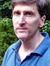# Greg Ross's Reviews > Mathematics 1001: Absolutely Everything That Matters in Mathematics in 1001 Bite-Sized Explanations

by's review

it was amazing

Popular books on mathematics tend to cover the same ground, highlighting a few easily explicated examples (Fibonacci's rabbits, Pascal's triangle, Hilbert's hotel) rather than trying to illuminate the whole landscape. Elwes has set out instead to create a concise layman's encyclopedia of mathematics, addressing each topic in clear language and including extensive cross-references to situate it in the larger picture. The result is an accessible, absorbing introduction to the discipline, touching on number theory, geometry, algebra, discrete math, analysis, logic, metamathematics, probability, mathematical physics, and some recreational math. The whole can be read straight through or consulted as a reference. One of the best "nonmathematical" surveys of math that I've seen.
flag